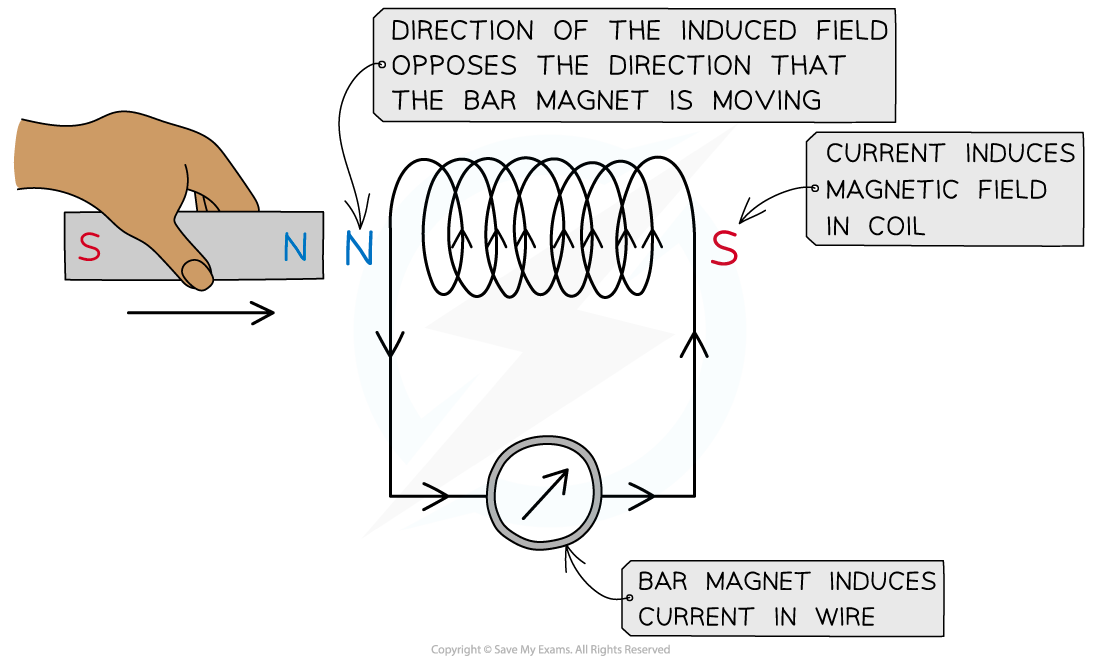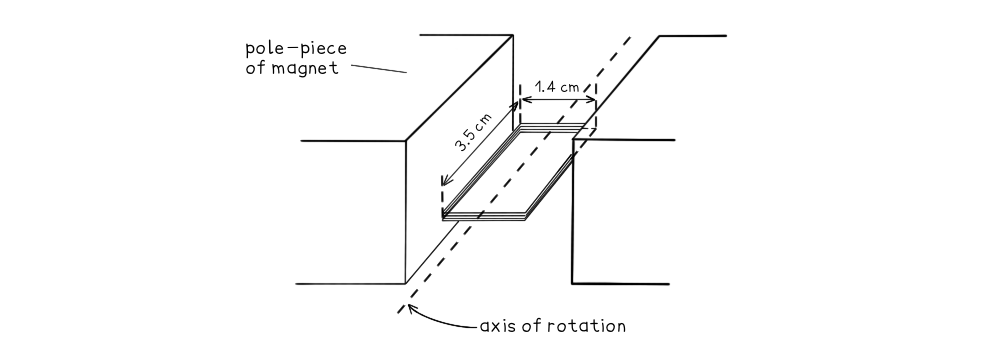# CIE A Level Physics复习笔记20.2.4 Faraday's & Lenz's Laws

### Faraday's & Lenz's Laws

• Faraday’s law tells us the magnitude of the induced e.m.f in electromagnetic induction and is defined as:

The magnitude of the induced e.m.f is directly proportional to the rate of change in magnetic flux linkage• Where:
• ε = induced e.m.f (V)
• N = number of turns of coil
• Δɸ = change in magnetic flux (Wb)
• Δt = time interval (s)
• Lenz’s Law gives the direction of the induced e.m.f as defined by Faraday’s law:

The induced e.m.f acts in such a direction to produce effects which oppose the change causing it

• Lenz’s law combined with Faraday’s law is:• This equation shows:
• When a bar magnet goes through a coil, an e.m.f is induced within the coil due to a change in magnetic flux
• A current is also induced which means the coil now has its own magnetic field
• The coil’s magnetic field acts in the opposite direction to the magnetic field of the bar magnet
• If a direct current (d.c) power supply is replaced with an alternating current  (a.c) supply, the e.m.f induced will also be alternating with the same frequency as the supply

#### Experimental Evidence for Lenz’s Law

• To verify Lenz’s law, the only apparatus needed is:
• A bar magnet
• A coil of wire
• A sensitive ammeter
• Note: a cell is not required
• A known pole (either north or south) of the bar magnet is pushed into the coil, which induces a magnetic field in the coil
• Using the right hand grip rule, the curled fingers indicate the direction of the current and the thumb indicates the direction of the induced magnetic field
• The direction of the current is observed on the ammeter
• Reversing the magnet direction would give an opposite deflection on the meter
• The induced field (in the coil) repels the bar magnet
• This is because of Lenz’s law:
• The direction of the induced field in the coil pushes against the change creating it, ie. the bar magnetLenz’s law can be verified using a coil connected in series with a sensitive ammeter and a bar magnet

#### Worked Example

A small rectangular coil contains 350 turns of wire. The longer sides are 3.5 cm and the shorter sides are 1.4 cm.The coil is held between the poles of a large magnet so that the coil can rotate about an axis through its centre.The magnet produces a uniform magnetic field of flux density 80 mT between its poles. The coil is positioned horizontally and then turned through an angle of 40° in a time of 0.18 s.Calculate the magnitude of the average e.m.f induced in the coil.

Step 1:            Write down the known quantities

Magnetic flux density, B = 80 mT = 80 × 10-3 T

Area, A = 3.5 × 1.4 = (3.5 × 10-2) × (1.4 × 10-2) = 4.9 × 10-4 m2

Number of turns, N = 350

Time interval, Δt = 0.18 s

Angle between coil and field lines, θ = 40o

Step 2:            Write out the equation for Faraday’s law:Step 3:            Write out the equation for flux linkage:

ɸN = BAN cos(θ)

Step 4:            Substitute values into flux linkage equation:

ɸN = (80 × 10-3) × (4.9 × 10-4) × 350 × cos(40) = 0.0105 Wb turns

Step 5:            Substitute flux linkage and time into Faraday’s law equation: